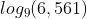## Log

Solve the shown logarithm:Hint
The logarithm of $$x$$ to the Base $$b$$ is defined by
$$log_b(x)=c$$$where $$b^c=x$$ . Hint 2 Factor 9 from 6,561. The logarithm of $$x$$ to the Base $$b$$ is defined by $$log_b(x)=c$$$
where $$b^c=x$$ .

The problem is asking to solve for $$c$$ :
$$9^c=6,561$$$Factor $$9$$ from $$6,561$$ : $$\frac{6,561}{9}=729\rightarrow \frac{729}{9}=81\rightarrow \frac{81}{9}=9$$$
$$9$$ evenly appears four times in $$6,561$$ . Thus,
$$log_9(9^4)=4$$\$
4